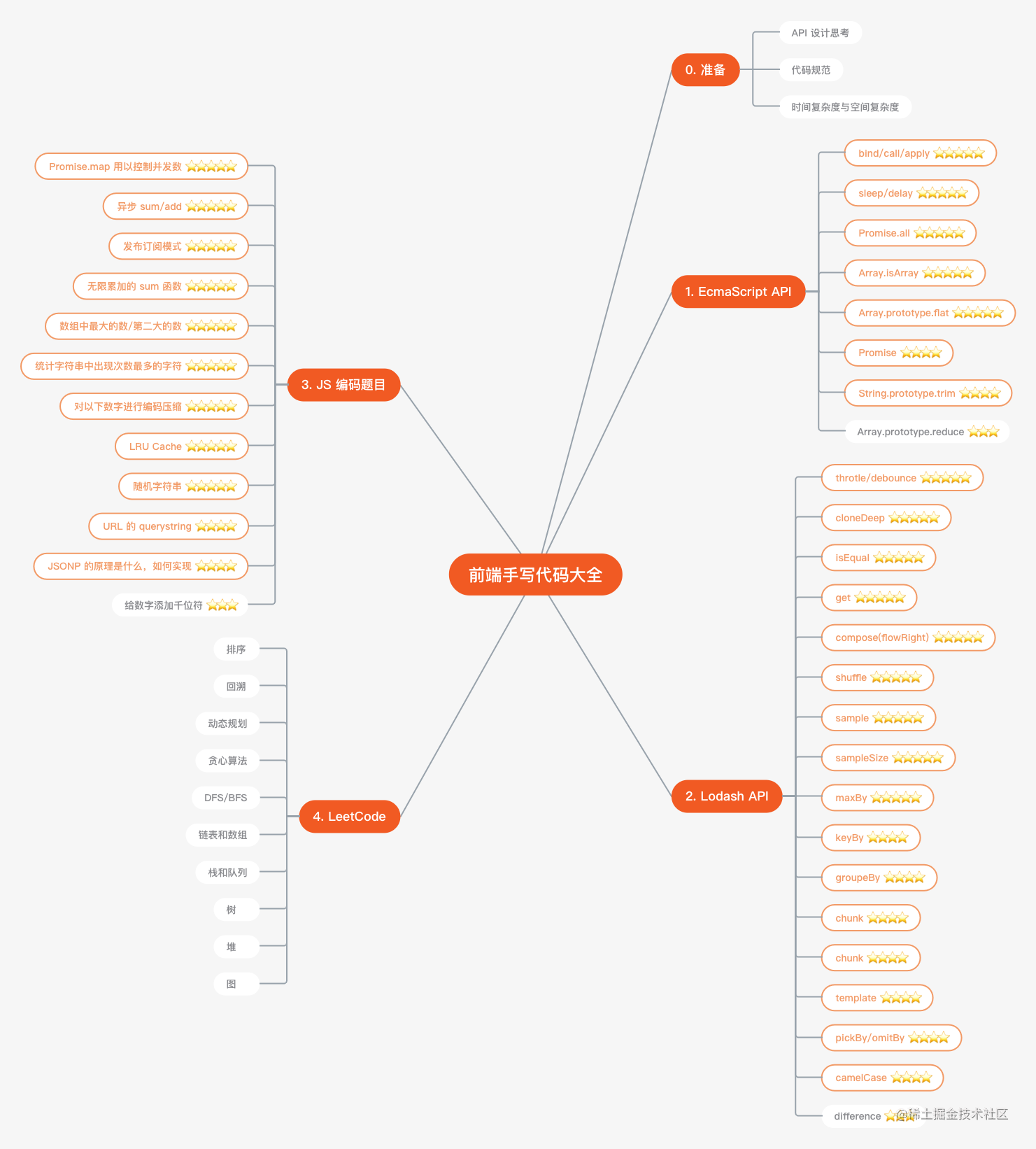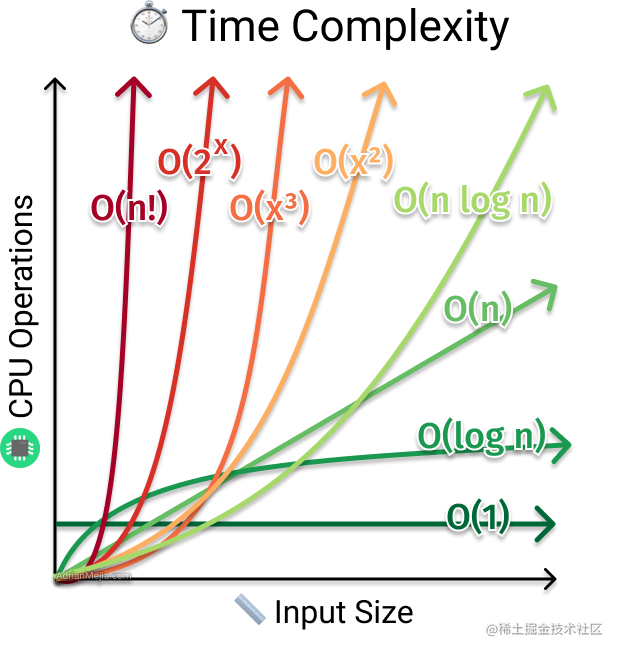# 面试大厂，应该练习那些手写代码## 准备工作

### API 设计思考

`compose` 函数常用在各种中间件设计中，如 `redux` 等。`redux` 函数的实现极为简单，甚至一行就能实现，但是能够第一个想到 `compose` 的更不容易。

### 代码规范

1. 是否有一致的代码规范
2. 是否有清晰可读的变量命名
3. 是否有更简介的代码

``````// BAD
// What the heck is 86400000 for?
setTimeout(blastOff, 86400000);

// GOOD
// Declare them as capitalized named constants.
const MILLISECONDS_PER_DAY = 60 * 60 * 24 * 1000; //86400000;

setTimeout(blastOff, MILLISECONDS_PER_DAY);``````

### 空间复杂度与时间复杂度

• 空间复杂度(Space Complexity) 描述该算法在运行过程中临时占用存储空间大小
• 时间复杂度(Time complexity) 描述该算法的运行时间，常用大O符号表述• O(1): Constant Complexity，常数复杂度，比如计算两次即可得出最终结论
• O(logn): Logarithmic Complexity，最常见的是二分查找
• O(n): Linear Complexity，一般为一个 for 循环，但半个或者两个 for 循环也视作 O(n)
• O(n^2): 一般为嵌套的 for 循环，如冒泡排序

## 手写代码路线图

1. ES API
2. lodash API
3. 编程逻辑题
4. 算法与数据结构 (leetcode)

## 01 ES API

### bind/call/apply ⭐⭐⭐⭐⭐️️️️

``````Function.prototype.fakeBind = function(obj, ...args) {
return (...rest) => this.call(obj, ...args, ...rest)
}``````

### sleep/delay ⭐⭐⭐⭐⭐

`sleep` 函数既是面试中常问到的一道代码题，也是日常工作，特别是测试中常用的一个工具函数。

``````const sleep = (seconds) => new Promise(resolve => setTimeout(resolve, seconds))

function delay (func, seconds, ...args) {
return new Promise((resolve, reject) => {
setTimeout(() => {
Promise.resolve(func(...args)).then(resolve)
}, seconds)
})
}``````

### Promise.all ⭐️⭐️⭐️⭐️⭐️

``````function pAll (_promises) {
return new Promise((resolve, reject) => {
// Iterable => Array
const promises = Array.from(_promises)
// 结果用一个数组维护
const r = []
const len = promises.length
let count = 0
for (let i = 0; i < len; i++) {
// Promise.resolve 确保把所有数据都转化为 Promise
Promise.resolve(promises[i]).then(o => {
// 因为 promise 是异步的，保持数组一一对应
r[i] = o;

// 如果数组中所有 promise 都完成，则返回结果数组
if (++count === len) {
resolve(r)
}
// 当发生异常时，直接 reject
}).catch(e => reject(e))
}
})
}``````

### Array.prototype.flat ⭐️⭐️⭐️⭐️⭐️

`reduce``concat` 简直是绝配

``````function flatten (list, depth = 1) {
if (depth === 0) return list
return list.reduce((a, b) => a.concat(Array.isArray(b) ? flatten(b, depth - 1) : b), [])
}``````

### Array.prototype.reduce ⭐️⭐️⭐️

``````const reduce = (list, fn, ...init) => {
let next = init.length ? init : list
for (let i = init.length ? 0 : 1; i < list.length; i++) {
next = fn(next, list[i], i)
}
return next
}``````

1. 回调函数中第一个 Index 是多少？
2. 数组为稀疏数组如何处理？

### String.prototype.trim ⭐️⭐️⭐️

``const trim = str => str.trim || str.replace(/^\s+|\s+\$/g, '')``

## 02 lodash API

### throtle/debounce ⭐⭐⭐⭐⭐️️

``````function throttle (f, wait) {
let timer
return (...args) => {
if (timer) { return }
timer = setTimeout(() => {
f(...args)
timer = null
}, wait)
}
}``````
``````function debounce (f, wait) {
let timer
return (...args) => {
clearTimeout(timer)
timer = setTimeout(() => {
f(...args)
}, wait)
}
}``````

### get ⭐️⭐️⭐️⭐️⭐️

``````function get (source, path, defaultValue = undefined) {
// a.b -> a.3.b -> [a, 3, b]
const paths = path.replace(/\[(\w+)\]/g, '.\$1').replace(/\["(\w+)"\]/g, '.\$1').replace(/\['(\w+)'\]/g, '.\$1').split('.')
let result = source
for (const p of paths) {
result = result?.[p]
}
return result === undefined ? defaultValue : result
}``````

### compose(flowRight) ⭐️⭐️⭐️⭐️⭐️

``````const compose = (...fns) =>
// 注意 f、g 的位置，如果实现从左到右计算，则置换顺序
fns.reduce((f, g) => (...args) => f(g(...args)))``````

### shuffle ⭐️⭐️⭐️⭐️⭐️

``const shuffle = (list) => list.sort((x, y) => Math.random() - 0.5)``

``````function shuffle (list) {
const len = list.length
let result = [...list]
for (let i = len - 1; i > 0; i--) {
const swapIndex = Math.floor(Math.random() * (i + 1));
[result[i], result[swapIndex]] = [result[swapIndex], result[i]]
}
return result
}``````

### sample ⭐️⭐️⭐️⭐️⭐️

Math.random() 函数返回一个浮点, 伪随机数在范围从0到小于1，用数学表示就是 [0, 1)，可以利用它来实现 sample 函数

``Array.prototype.sample = function () { return this[Math.floor(Math.random() * this.length)] }``

### sampleSize ⭐️⭐️⭐️⭐️⭐️

``````const shuffle = (list) => list.sort((x, y) => Math.random() - 0.5)
const sampleSize = (list, n) => shuffle(list).slice(0, n)``````

### chunk ⭐️⭐️⭐️⭐️

``````function chunk (list, size) {
const l = []
for (let i = 0; i < list.length; i++ ) {
const index = Math.floor(i / size)
l[index] ??= [];
l[index].push(list[i])
}
return l
}``````

### chunk ⭐️⭐️⭐️⭐️

``````const f = x => x

const onceF = once(f)

//=> 3
onceF(3)

//=> 3
onceF(4)``````

### template ⭐⭐⭐⭐️️️️️

``````const template = '{{ user["name"] }}，今天你又学习了吗 - 用户ID: {{ user.id }}';

const data = {
user: {
id: 10086,
name: '山月',
}
};

//=> "山月，今天你又学习了吗 - 用户ID: 10086"
render(template, data); ``````

1. 注意深层嵌套数据
2. 注意 `user['name']` 属性

## 03 编程逻辑题

### FizzBuzz，是否能被3或5整除 ⭐️⭐️⭐️⭐️⭐️

``````//=> 'fizz'
fizzbuzz(3)

//=> 'buzz'
fizzbuzz(5)

//=> 'fizzbuzz'
fizzbuzz(15)

//=> 7
fizzbuzz(7)``````

### 如何实现无限累加的 sum 函数 ⭐️⭐️⭐️⭐️⭐️

``````sum(1, 2, 3).valueOf() //6
sum(2, 3)(2).valueOf() //7
sum(1)(2)(3)(4).valueOf() //10
sum(2)(4, 1)(2).valueOf() //9
sum(1)(2)(3)(4)(5)(6).valueOf() // 21``````

• sum 返回一个函数，收集所有的累加项，使用递归实现
• 返回函数带有 valueOf 属性，用于统一计算
``````function sum (...args) {
const f = (...rest) => sum(...args, ...rest)
f.valueOf = () => args.reduce((x, y) => x + y, 0)
return f
}``````

### 统计数组中最大的数/第二大的数 ⭐️⭐️⭐️⭐️⭐️

``````function max (list) {
if (!list.length) { return 0 }
return list.reduce((x, y) => x > y ? x : y)
}``````

``````function maxTwo (list) {
let max = -Infinity, secondMax = -Infinity
for (const x of list) {
if (x > max) {
secondMax = max
max = x
} else if (x > secondMax) {
secondMax = x
}
}
return [max, secondMax]
}``````

### 统计字符串中出现次数最多的字符 ⭐️⭐️⭐️⭐️⭐️

``````function getFrequentChar (str) {
const dict = {}
for (const char of str) {
dict[char] = (dict[char] || 0) + 1
}
const maxBy = (list, keyBy) => list.reduce((x, y) => keyBy(x) > keyBy(y) ? x : y)
return maxBy(Object.entries(dict), x => x)
}``````

``````function getFrequentChar2 (str) {
const dict = {}
let maxChar = ['', 0]
for (const char of str) {
dict[char] = (dict[char] || 0) + 1
if (dict[char] > maxChar) {
maxChar = [char, dict[char]]
}
}
return maxChar
}``````

### 对以下数字进行编码压缩 ⭐️⭐️⭐️⭐️⭐️

• Input: 'aaaabbbccd'
• Output: 'a4b3c2d1'，代表 a 连续出现四次，b连续出现三次，c连续出现两次，d连续出现一次

``````//=> a4b3c2
encode('aaaabbbcc')

//=> a4b3a4
encode('aaaabbbaaaa')

//=> a2b2c2
encode('aabbcc')``````

• 如果只出现一次，不编码数字，如 aaab -> a3b
• 如果只出现两次，不进行编码，如 aabbb -> aab3
• 如果进行解码数字冲突如何解决

``````function encode (str) {
const l = []
let i = 0
for (const s of str) {
const len = l.length
const lastChar = len > 0 ? l[len - 1] : undefined
if (lastChar === s) {
l[len - 1]++
} else {
l.push([s, 1])
}
}
return l.map(x => x.join('')).join('')
}``````

``````> encode('aaab')
< "a3b1"``````

1. 如果只出现一次，不编码数字，如 `aaab -> a3b`
2. 如果只出现两次，不进行编码，如 `aabbb -> aab3`
3. 如果进行解码，碰到数字如何处理？

``````function encode (str) {
const l = []
let i = -1;
let lastChar
for (const char of str) {
if (char !== lastChar) {
lastChar = char
i++
l[i] = [char, 1];
} else {
l[i]++
}
}
return l.map(([x, y]) => {
if (y === 1) {
return x
}
if (y === 2) {
return x + x
}
return x + y
}).join('')
}``````

### JSONP 的原理是什么，如何实现 ⭐️⭐️⭐️⭐️

`JSONP`，全称 `JSON with Padding`，为了解决跨域的问题而出现。虽然它只能处理 GET 跨域，虽然现在基本上都使用 CORS 跨域，但仍然要知道它，毕竟面试会问

`JSONP` 基于两个原理:

1. 动态创建 `script`，使用 `script.src` 加载请求跨过跨域
2. `script.src` 加载的脚本内容为 JSONP: 即 `PADDING(JSON)` 格式
``````function jsonp ({ url, onData, params }) {
const script = document.createElement('script')

// 一、为了避免全局污染，使用一个随机函数名

// 二、默认 callback 函数为 cbFnName
script.src = `\${url}?\${stringify({ callback: cbFnName, ...params })}`

// 三、使用 onData 作为 cbFnName 回调函数，接收数据
window[cbFnName] = onData;

document.body.appendChild(script)
}

// 发送 JSONP 请求
jsonp({
url: 'http://localhost:10010',
params: { id: 10000 },
onData (data) {
console.log('Data:', data)
}
})``````

### 使用 JS 如何生成一个随机字符串 ⭐️⭐️⭐️⭐️⭐️

``const random = (n) => Math.random().toString(36).slice(2, 2 + n)``

### 给数字添加千位符 ⭐️⭐️⭐️

``````function numberThousands (number, thousandsSeperator = ',') {
return String(number).replace(/(\d)(?=(\d\d\d)+(?!\d))/g, '\$1' + thousandsSeperator)
}``````

## 04 算法与数据结构 (leetcode)

Leetcode 简单与中级难度题目 200/100 道，以简单题目为主。直接刷就得了。

### TopK 问题

1. 取数组中前 k 个数做小顶堆，堆化
2. 数组中的其它数逐一与堆顶元素比较，若大于堆顶元素，则插入该数

### 求给定数组中 N 个数相加之和为 sum 所有可能集合

``function fn(arr, n, sum) {}``